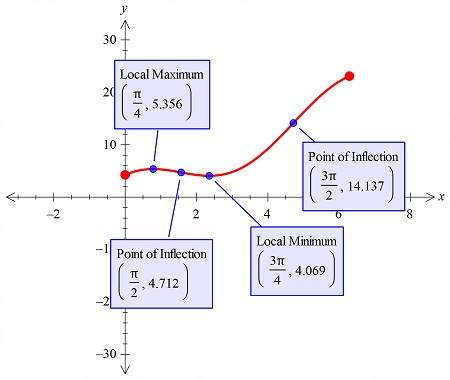# Graph the function by determining the features of the curve represented by y = 3x + 3\sqrt{2}\cos...

## Question:

Graph the function by determining the features of the curve represented by

{eq}y = 3x + 3\sqrt{2}\cos x; \quad 0 \leq x \leq 2x {/eq}.

Identify any local and absolute extreme points and inflection points.

## Graphs:

For the equation in two variables, we can have the maxima or the minima of the value, if we are given the domain interval of the function. the graphs are plotted and these points are shown.

## Answer and Explanation:

To find the extrema and the inflection points, we will use the graphical method.

So, the curve is plotted in the figure below:We have shown all the extreme points and the two inflection points in the given interval.

#### Learn more about this topic:Graphs: Types, Examples & Functions

from High School Algebra II: Help and Review

Chapter 16 / Lesson 11
355K